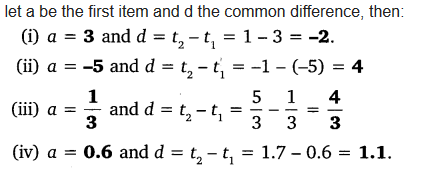# NCERT/CBSE MATHS Class 10 Ex-5.1 Q No.3 Solutions : A.P.s write the first term and the common difference

0
48# NCERT/CBSE MATHS Class 10 Ex-5.1 Q No.3 Solutions : For the following A.P.s, write the first term and the common difference.

Hy Friends Welcome On NCERT MATHS SOLUTIONS !! Today we are going to solve the Question : For the following A.P.s, write the first term and the common difference.of Exercise 5.1 Question No 3 Solutions (Arithmetic Progressions) of Class 10th, which will prove to be very helpful for you.

# CBSE/NCERT MATHS Class 10 Ex-5.1 Q No. 3 Solutions

If you are a student of CBSE, today we are going to give you the CBSE / NCERT Chapter : Arithmetic Progressions Exercise 5.1 Question No – 3 Solutions . Hope you like this post about Class 10th Maths Solution.

Here we would like to tell you that there are other websites on which the students demanded the Solution of NCERT MATHS Chapters, we had promised them that we will soon send you the questions of Class 9, And we completed our promise to them.We asked the good knowledgeable teachers of mathematics and asked them to solve the issues of CBSE’s mathematical questions and also said that the answers to our questions are such that the students have no difficulty in solving all those questions.

### Arithmetic Progressions CHAPTER : 5

n this progression, for a given series, the terms used are the first term, the common difference between the two terms and nth term.

Suppose, a1, a2, a3, ……………., an is an AP, then; the common difference “ d ” can be obtained as; In this progression, for a given series, the terms used are the first term, the common difference between the two terms and nth term.

a, a + d, a + 2d, a + 3d, a + 4d, ………. ,a + (n – 1) d

### For the following A.P.s, write the first term and the common difference.

(i) 3, 1, – 1, – 3 …
(ii) -5, – 1, 3, 7 …
(iii) 1/3, 5/3, 9/3, 13/3 ….
(iv) 0.6, 1.7, 2.8, 3.9 …

### Class 10 Exercise 5.1 Question No. 3 Solutions

(i) 3, 1, – 1, – 3 …
Here, first term, a = 3
Common difference, d = Second term – First term
= 1 – 3 = – 2

(ii) – 5, – 1, 3, 7 …
Here, first term, a = – 5
Common difference, d = Second term – First term
= ( – 1) – ( – 5) = – 1 + 5 = 4

(iii) 1/3, 5/3, 9/3, 13/3 ….
Here, first term, a = 1/3

Common difference, d = Second term – First term

= 5/3 – 1/3 = 4/3

(iv) 0.6, 1.7, 2.8, 3.9 …
Here, first term, a = 0.6
Common difference, d = Second term – First term
= 1.7 – 0.6
= 1.1

## OR

### Solution:About NCERT Maths Solutions :-

यहाँ पर हम आपको बताना चाहेंगे कि हमारी अन्य websites भी हैं जिन पर छात्रों ने NCERT MATHS Chapters के Solution की काफी मांग की थी , हमने उनसे वादा किया था कि हम जल्द ही आपको Class 9th ,10th  के गणित के सवालों के हल करके आप तक पहुचाएंगे और हमने उनसे किया अपना वादा पूरा किया |
हमने गणित के अच्छे जानकार ढूंढे और उनसे CBSE के गणित के सवालों के आसन हल करने को कहा साथ ही यह बात भी कही की हमारे सवालों के जवाब कुछ ऐसे हो जिनमे छात्रों को उन सभी सवालों को हल करने में कोई कठिनाई न हो |

If you have any question from NCERT, then you can ask your question here in the comment box. We will be happy to answer your questions.